# Abscissas and Weights of Classical Gaussian Quadrature Rules

by on May 30, 2012

The latest version of the Multiprecision Computing Toolbox introduces new routines to compute the nodes and weights of classical Gaussian quadrature rules with any required accuracy. A summary of the supported functionality is outlined below.

The Gaussian quadrature is targeted to approximate an integral by taking the weighted sum of integrand values sampled at special points (called abscissas). The abscissas and weights are calculated in a special way so that the rule provides a precise answer for all polynomials up to certain degree.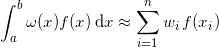The most common case, the Gauss-Legendre quadrature, occurs when the weight function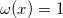. However if the integrand has a factor (weight) of a special form, then a more efficient quadrature can be applied. This is beneficial for both accuracy and computational speed.

The table below lists the classical Gaussian quadrature rules with corresponding weight functions and parameters. Note that the formulas are provided in generalized form, already adjusted for the arbitrary intervals of integration.

NameWeight function,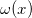IntervalFunction
Legendre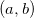mp.GaussLegendre
Chebyshev Type 1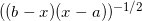mp.GaussChebyshevType1
Chebyshev Type 2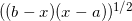mp.GaussChebyshevType2
Gegenbauer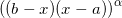mp.GaussGegenbauer
Jacobi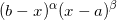mp.GaussJacobi
Generalized Laguerre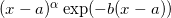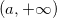mp.GaussLaguerre
Generalized Hermite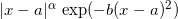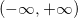mp.GaussHermit

The last column in the table shows the functions provided by the Multiprecision Computing Toolbox for computing abscissas and weights for each corresponding quadrature with arbitrary precision. A detailed description of the functions and parameters is available using the standard MATLAB’s command help:

>> help mp.GaussLaguerre mp.GaussLaguerre - Arbitrary precision coefficients of Generalized Gauss-Laguerre Quadrature   [x,w] = mp.GaussLaguerre(order, alpha, a, b) computes abscissas 'x' and weights 'w' of Generalized Gauss-Laguerre quadrature.   The generalized Gauss-Laguerre quadrature rule is used as follows:   Integral ( a < x < +inf ) (x-a)^alpha * exp(-b*(x - a)) f(x) dx   is to be approximated using 'x' and 'w' by   sum ( 1 <= i <= order ) w(i) * f(x(i))   Parameters: 'order' is the number of points in the quadrature rule. 'alpha' is the exponent of |x| in the weight function. The value of alpha may be any real value greater than -1.0. Specifying alpha = 0.0 (default) results in the basic (non-generalized) rule. 'a' is the left point (default 0); 'b' is the scale factor in the exponential (default 1);   Precision is controlled by mp.Digits and by precision of the arguments

For example, let’s compute the 10th order Gauss-Laguerre quadrature rule to an accuracy of 50 decimal digits with the default parameters (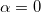,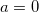,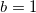):

>> mp.Digits(50); >> [x,w] = mp.GaussLaguerre(10); >> x 0.13779347054049243083077250565271118810799168074578 0.72945454950317049816037312167607878107607273331225 1.80834290174031604823292007575060883328306028237145 3.40143369785489951448253222140839067927315661420347 5.55249614006380363241755848686876285797406428731781 8.33015274676449670023876719727452218270943897203099 11.84378583790006556491853891914161398580281690946534 16.27925783137810209953265393583362233525599560303306 21.99658581198076195127709019559449397680673234000189 29.92069701227389155990879334079919517971067057751796   >> w 0.30844111576502014154747083467786069562872888653834 0.40111992915527355151578030991281951479548361696211 0.21806828761180942158864852347464672674277853841219 0.06208745609867774739290212931351795369590906568380 0.00950151697518110055383907219417199122586245040158 0.00075300838858753877545596435367566390179203914014 0.00002825923349599565567422563826850021282803316474 0.00000042493139849626863725865766597471235464810802 0.00000000183956482397963078092153522435593824798261 0.00000000000099118272196090085583775472832447360646

Example 1. The Runge function integral approximation on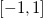using the Gauss-Legendre quadrature: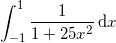>> mp.Digits(50); >> f = @(x) 1./(1+25.*x.^2); >> [x,w] = mp.GaussLegendre(50,-1,1); % GaussLegendre(order, a, b) >> sum(f(x).*w) 0.54936030435978284812028223550277229574990724174503

Example 2. The Gauss-Laguerre rule can be used to compute values of Gamma function: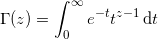The function under the integral corresponds to the Laguerre weight with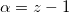,,, and the approximation can be calculated as ():

>> mp.Digits(50); >> z = mp('pi'); >> [t,w] = mp.GaussLaguerre(30, z-1); >> sum(w) 2.28803779534003241795958890906023392288968815335622   >> gamma(z) 2.28803779534003241795958890906023392288968815335622

## Implementation

The toolbox uses the Golub-Welsch method, to compute all Gaussian quadrature abscissas and weights.

At first we construct a tri-diagonal Jacobi matrix using coefficients of the recurrence relation of orthogonal polynomials built with theweight function on the required interval. The quadrature’s nodes are eigenvalues of the Jacobi matrix, and weights are computed from the first components of the corresponding eigenvector. Efficient, implicit QL decomposition is used to find the eigenvalues and eigenvectors.

The code of the procedure:

 % Build tri-diagonal Jacobi matrix [aj, bj, mu] = jacobi_matrix(kind, N, alpha, beta);   J = diag(aj,0) + diag(bj(1:N-1),1) + diag(bj(1:N-1),-1);   % Find eigenvectors & eigenvalues of tridiagonal Jacobi matrix % This gives us quadrature rules for default intervals [V,D] = eig(J); [x,i] = sort(diag(D)); w = mu * V(1,i)'.^2;

Toolbox’s implementation was inspired by the MATLAB code of John Burkardt, which is based on the FORTRAN library of Sylvan Elhay and Jaroslav Kautsky,.

Additional information on the Gaussian quadrature and numerical integration can be found at  and .

## References

1. Golub G., Welsch J., Calculation of Gauss quadrature rules, Math. Соmр. 23 1969.^
2. Gautschi W., Orthogonal Polynomials: Computation and Approximation. Oxford University Press. 2004.^
3. Martin R., Wilkinson J., The Implicit QL Algorithm, Numerische Mathematik, Volume 12, Number 5, December 1968, pages 377-383.^
4. Burkardt, J., Weights for Interpolatory Quadrature. ^
5. Elhay S., Kautsky J., Calculation of the Weights of Interpolatory Quadratures, Numerische Mathematik, Volume 40, 1982, pages 407-422.^
6. Elhay S., Kautsky J., Algorithm 655: IQPACK, FORTRAN Subroutines for the Weights of Interpolatory Quadrature, ACM Transactions on Mathematical Software, Volume 13, Number 4, December 1987, pages 399-415.^
7. Davis P., Rabinowitz P., Methods of Numerical Integration, Second Edition, Dover, 2007, ISBN: 0486453391, LC: QA299.3.D28.^
8. Stroud A., Secrest D., Gaussian Quadrature Formulas, Prentice Hall, 1966, LC: QA299.4G3S7.^